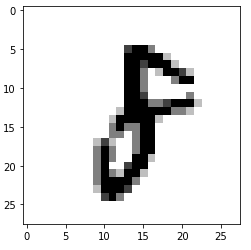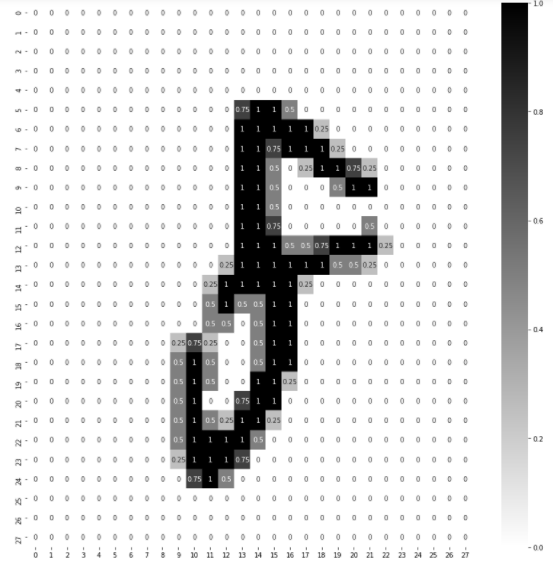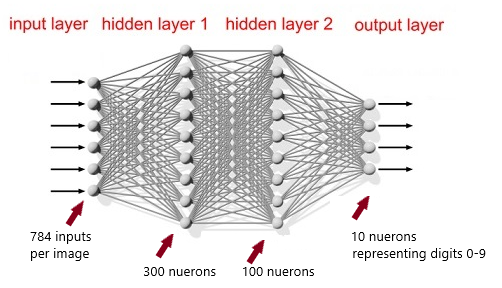# Deep Learning — ‘Hello World!’

This is a continuation from my previous article. If you haven’t read that, I strongly suggest you to read that first to get a high level overview of Deep Learning concepts. Here is the link to Part 1 (Deep Learning — Beginners Guide)

I could have dived deep into more theory and math part of activation functions, loss functions, optimizers etc. But I thought, Its a good idea to get our hands dirty and implement the first Artificial Neural Network (ANN) to get an understanding of the tool set. So let get started.

# Installing Software and Setting up Environment

To begin with, you will need to have Anaconda distribution installed on your computer. If you haven’t yet, go ahead and download from Anaconda site. Once installed, you need to setup the environment. A conda environment is typically a set of related software’s which are compatible with each other. When you create a environment, anaconda creates a directory and stores all the relevant software’s for that environment in that directory. Follow the below steps to create the environment.

1. Open the Anaconda prompt (default environment is shown as (base)) and type the below:
`conda create -n helloworld python==3.6.9conda activate helloworld`

You can find the cheetsheet for different conda commands here.

2. Once the environment is created and activated, you need to install the below softwares which are needed for our first ANN implementation.

`pip install jupyterpip install pandaspip install matplotlibpip install seabornpip install tensorflow`

A quick introduction to above softwares.

Jupyter Notebook allows you to write live code and share the notebooks with others. You will be using it a lot going forward.

Pandas is a python data analysis library. The key data structure is a DataFrame.

Matplotlib and Seaborn are data visualization libraries and are very effective for exploratory data analysis.

Tensorflow is a machine learning and deep learning library developed by Google. By default, it will install version 2 of tensorflow. Note that there are slight differences b/w version 1 and version 2 of tensorflow. I suggest, you work with latest version.

Keras provides high level APIs and comes integrated with tensorflow 2. Its a API wrapper built on top of tensorflow.

3. Once all the softwares are installed, from the anaconda prompt, type `jupyter notebook`. This will open up a browser window. On the top right, there will be a “New” drop-down. Select “Python 3” from the drop-down. From the File menu, select “Save as” and save the notebook as “hello world”. you can check the version of tensorflow and Keras using the below statements.

`import tensorflow as tfprint(f"tensorflow version: {tf.__version__}")print(f"Keras version: {tf.keras.__version__}")`

If you have come this far, the plumbing work is complete 😌 We are now ready to implement our first ANN. But first, lets look at the MNIST dataset.

# MNIST Dataset

The MNIST dataset contains 60,000 training images and 10,000 testing images. The dataset contains hand written digits from 0 to 9. Each data point is a 2D array of 28 X 28 size. We will use this dataset to train our model to predict hand written digits. Here is the visual image of the dataset (source: wikipedia)

# Our First ANN Implementation

Now, we are ready to go 😃 I just want you to know that there might be still few things which may not be well understood at this moment. I will try to write in brief about them. They will be covered in much more detail in upcoming articles. The intention here is to introduce you to the world of deep learning tool set and how to use them.

Load the libraries and import MINST dataset: The first step is to load libraries and import dataset. MNIST dataset is now bundled as part of Keras datasets. Below is the code to do this:

`import numpy as npimport matplotlib.pyplot as pltimport pandas as pdimport osimport tensorflow as tfimport seaborn as sns# Step 1. Load train and test data set.mnist = tf.keras.datasets.mnist(X_train_full, y_train_full), (X_test, y_test) = mnist.load_data() # Step 2. check the size of training and test datasets print(X_train_full.dtype, "-", X_train_full.shape)print(y_train_full.dtype, "-", y_train_full.shape)print(X_test.dtype, "-", X_test.shape)print(y_test.dtype, "-", y_test.shape)# Step 3. Randomly check one of the data points.X_train_fully_train_full`

The code is self explanatory and nothing complicated. When you execute `X_train_full,` it will display a 2D array of 28 X 28 with numbers ranging b/w 0 and 255, since each data point is 28 X 28 size. The `dtype` is `unit8` which holds values b/w 0 and 255.

Scale the data and create validation set: The next step is to scale the data b/w 0 and 1 and create the validation dataset. For the validation dataset, we will divide the `X_train_full, y_train_full` into two sets of `X_valid, X_train` and `y_valid, y_train`.

`# Scale the data b/w 0 and 1 by dividing it by 255 as its unsigned intX_train_full = X_train_full/255.X_test = X_test/255.# View the matrix now. The values will be b/w 0 and 1 X_train_full# Create the validation data from training data.X_valid, X_train = X_train_full[:5000], X_train_full[5000:]y_valid, y_train = y_train_full[:5000], y_train_full[5000:]X_train.shape# should give o/p of (55000, 28, 28)X_valid.shape# should give o/p of (5000, 28, 28)`

We have a validation set of 5000 records and training set of 55000 records. By the way, we haven’t used matplotlib and seaborn until now. Let us use them to see the images in jupyter notebook. Execute the below code to see the output of the actual image and also the heatmap for the image.

`# view the actual image at index 30plt.imshow(X_train, cmap='binary')`

The output of the above will be as below:Figure 2: visual image at index 30
`# Lets look at the pixels in detail using SNSplt.figure(figsize=(15,15))sns.heatmap(X_train, annot=True, cmap='binary')`

And this code shows you the complete 28 X 28 grid with data of each pixel as below.Figure 3: Pixel view of the image

Model Building: Its now time to build our model. The concepts from my first article will be useful to understand it better. Here is the code to build the model.

`# lets create the model# Flatten = make the array to sequential layer# Dense = creating a hidden OR output layerLAYERS = [tf.keras.layers.Flatten(input_shape=[28,28],name="inputLayer"),         tf.keras.layers.Dense(300, activation="relu", name="hiddenLayer1"),         tf.keras.layers.Dense(100, activation="relu", name="hiddenLayer2"),         tf.keras.layers.Dense(10, activation="softmax", name="outputLayer")]model = tf.keras.models.Sequential(LAYERS)`

A lot is happening here. Let me explain

Input Layer: We have flattened the input matrix of 28 X 28. Which means we will have 28 x 28 = 784 input values per image.

Hidden Layers: We use ‘Dense’ to create hidden and output layers. In the above code, we have created 2 hidden layers. We have 300 and 100 neurons in hidden layers 1 and 2. These are just random values I picked. You can chose any other values as of now. Later in my tutorials, I will show how to arrive at these values using Keras tuner. I am using ‘relu’ activation function in the hidden layers. Again, just follow this as of now. We will discover more on activation functions as we learn about them.

Output Layer: The output layer is having 10 neurons since we have values b/w 0 and 9 in our dataset. We are using ‘softmax’ as activation in output layer since we are dealing with multi-class classification problem.

You can imagine our neural network as below:Figure 4: Deep neural network with 2 hidden layers

Let us now look at the summary of the model. It has a lot of information to be digested. Execute the code `model.summary()` in jupyter notebook. You should see the below output.

It shows 3 columns. “Layer” column shows the name of the layer. “Output Shape” column shows number of neurons in each layer. “Param #” is the column which needs to be understood. There are some random numbers in there. Let me explain.

Param # is the calculation of weights and biases. In hiddenLayer1, we have 300 neurons receiving inputs from 784 neurons from inputLayer. Which means that we have 300 X 784 = 235200 weights. Biases are equal to number of neurons in that layer. In hiddenLayer1, its 300. So if you add up weights and biases you get 235500 (235200 + 300). Similarly the values for other layers can be calculated as below:

`# Param # (Nodes in layer A * Nodes in layer B + Bias)# hiddenLayer1 = 784*300 + 300 = 235500# hiddenLayer2 = 300*100 + 100 = 30100# outputLayer = 100*10 + 10 = 1010# Trainable Params = 235500 + 30100 + 1010 = 266610`

Trainable Params is the total number of weights and biases which can be modified to train the model. If you add up above numbers, you get 266,610. I hope this is crystal clear now. Lets move on.

Weights and Biases: Let us look at the weights and biases. We can use the below code to view the weights and biases which are assigned initially.

`hidden1 = model.layersweights, biases = hidden1.get_weights()#weights should be a metrics of 784 X 300 and biases should be 300weights.shapebiases.shapeprint(weights)print(biases)`

When you execute the print statements, you will see random values for weights and 0 values for all biases. These are updated as the model starts learning during back propagation.

Loss function, Optimizer for back propagation: We now need to define the operations for back propagation. We need to set the loss function to be used, optimizer for updating weights and biases and the metrics for accuracy.

`LOSS_FUNCTION = "sparse_categorical_crossentropy"OPTIMIZER = "SGD"METRICS = ["accuracy"]model.compile(loss=LOSS_FUNCTION,             optimizer=OPTIMIZER,             metrics=METRICS)`

I am using the `sparse_catagorical_crossentropy` as the loss function and `stochastic gradient descent` as the optimizer. I will write more about these in future articles. The metrics specifies the parameter which we want to use as a measure to evaluate the model performance. Once all these are chosen, we call compile method on the model.

Model Training: Its time to train our model and see the how well its performing. We need to understand one new term and its called EPOCH. Simply put, epoch is the number of times model has to be evaluated during training.

`EPOCHS = 30VALIDATION_SET = (X_valid, y_valid)history = model.fit(X_train, y_train, epochs=EPOCHS,                   validation_data=VALIDATION_SET)`

In the code above, we have defined 30 epochs, which means that the model has to do forward propagation and back propagation 30 times. The validation set is used to validate out model against training data set. When the code is executed, you will see output something as below:

`Epoch 1/301719/1719 [==============================] - 5s 3ms/step - loss: 0.6110 - accuracy: 0.8479 - val_loss: 0.3095 - val_accuracy: 0.9162Epoch 2/301719/1719 [==============================] - 5s 3ms/step - loss: 0.2867 - accuracy: 0.9175 - val_loss: 0.2354 - val_accuracy: 0.9360Epoch 3/301719/1719 [==============================] - 5s 3ms/step - loss: 0.2328 - accuracy: 0.9341 - val_loss: 0.2017 - val_accuracy: 0.9450Epoch 4/301719/1719 [==============================] - 6s 3ms/step - loss: 0.1986 - accuracy: 0.9433 - val_loss: 0.1725 - val_accuracy: 0.9506............Epoch 30/301719/1719 [==============================] - 6s 3ms/step - loss: 0.0285 - accuracy: 0.9937 - val_loss: 0.0681 - val_accuracy: 0.9808`

batch size and no. of batches: When the model is being trained, it doesn’t pass one input every iteration. Instead, it take a batch size. The `fit` method has a batch_size parameter, which by default is 32 if not specified. So in our case, considering a batch size of 32 and a training set of 55000, we get the number of batches to be 1719.

Below is the brief of all the parameters of the output:

`# Epoch 1/30# 1719/1719 [==============================] - 5s 3ms/step - loss: 0.6110 - accuracy: 0.8479 - val_loss: 0.3095 - val_accuracy: 0.9162# default batch size=32# No. of batches = X_train.shape/batch_size = 55000/32 = 1719# 1719 = No of batches# 5s = 5 seconds for one single Epoch# 3ms/step = time taken for one batch# loss: 0.6110 = training loss (summation of all losses in all batches)# accuracy: 0.8479 = training accuracy (summation for all batches)# val_loss: 0.3095 = validation loss# val_accuracy: 0.9162 = validation accuracy`

When you observe the output of model training, you can see the accuracy improving after every epoch. Which suggests that the model is learning by adjusting weights and biases which are the trainable parameters. We can visually see how the model reduced loss and increased accuracy using the `history` we captured during model training. Here is the code and visual representation

`pd.DataFrame(history.history).plot(figsize=(8,5))plt.grid(True)plt.gca().set_ylim(0,1)plt.show()`

From the above figure, its clear that after 20 epochs (x-axis), the model is not learning much. We have ways to optimize and we will discuss on how to do that in upcoming articles.

Model Testing: Now let us test our model against the test data we created at the beginning and see how it performs. The code to do this is straight forward.

`# validate against test data nowmodel.evaluate(X_test, y_test)#Output:#313/313 [==============================] - 1s 2ms/step - loss: #0.0734 - accuracy: 0.9763`

As we can see from the output, the loss and accuracy are very much close to validation dataset (val_loss: 0.0681 — val_accuracy: 0.9808). We could tune this is bit more but out of scope for this article.

Let us now take some sample from test data set and try to see if we get the right predictions:

`X_new = X_test[:3]y_pred = np.argmax(model.predict(X_new), axis=-1)y_test_new = y_test[:3]for data, pred, actual in zip(X_new, y_pred, y_test_new):    plt.imshow(data, cmap="binary")    plt.title(f"Predicted: {pred}, Actual: {actual}")    plt.axis('off')    plt.show()    print("---"*20)`

We are taking the first 3 values from test data and trying to predict the values. We are then comparing the predicted values v/s actual values in the loop. I got all the 3 predictions correct. You can try out with some other random samples OR by converting some of the other hand written images into 28 X 28 pixel and see how this model performs.

This completes our ‘hello world’ for deep learning. Before closing this article, I want to showcase one more tool which can be very handy to visually examine out model. The tool is called ‘Netron’. You can either download it from the github location OR open your saved model directly in browser window using the URL: https://netron.app/. I will leave you to explore more on this tool which comes in quite handy for initial learning.

You can refer the jupyter notebook for the MNIST implementation here.

Hmm… If you have come till this line and understood at least 40% of the article, you have achieved what is need to go ahead. Keep learning.

--

--